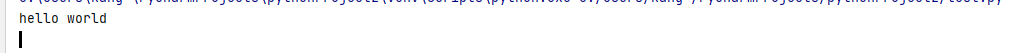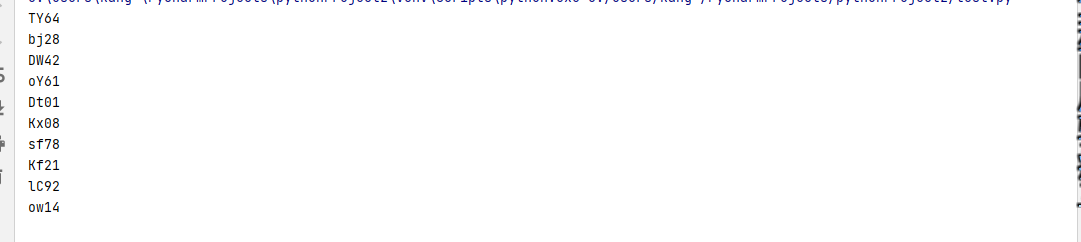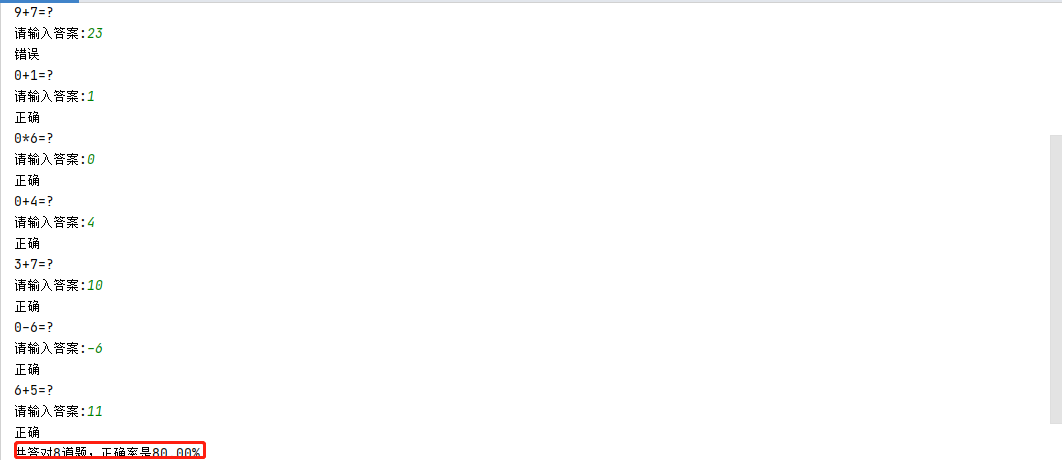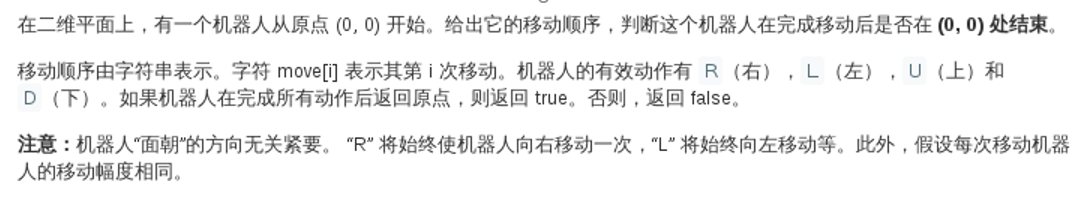## Python字符串的用法

2021/2/6 23:54:18 文章标签: 测试文章如有侵权请发送至邮箱809451989@qq.com投诉后文章立即删除

python字符串的用法1 Python常用转义字符2 字符串的基本用法2.1 连接操作符和重复操作符2.2 成员操作符2.3 正向索引和反向索引2.4 切片2.5 for循环遍历字符串3 python 字符串常用操作方法3.1 数据清洗3.2 字符串的类型判断3.3 字符串类型的转换3.4 判断字符串的开头的和结尾3.…

### python字符串的用法

• 1 Python常用转义字符
• 2 字符串的基本用法
• 2.1 连接操作符和重复操作符
• 2.2 成员操作符
• 2.3 正向索引和反向索引
• 2.4 切片
• 2.5 for循环遍历字符串
• 3 python 字符串常用操作方法
• 3.1 数据清洗
• 3.2 字符串的类型判断
• 3.3 字符串类型的转换
• 3.4 判断字符串的开头的和结尾
• 3.5 字符串位置的调整
• 3.6 字符串的搜索和统计
• 3.7 字符串的分离和拼接
• 4 随机生成数字和字母
• 4.1 随机生成数字
• 4.2 随机生成字符
• 4.3 随机生成数字和字母的组合
• 5 测试题

# 1 Python常用转义字符

\ 在行尾时是续行符
\ \反斜杠符号
\ ’单引号
\ "双引号
\a响铃
\b退格
\e转义
\000
\n换行
\v纵向制表符
\t横向制表符
\r回车
\f换页
\oyy八进制数，yy代表字符，如 \o12 代表换行
\xyy十六进制数 yy代表字符，如\x0a代表换行
\other其他字符以普通格式输出

# 2 字符串的基本用法

## 2.1 连接操作符和重复操作符

（1）连接操作符 “+”

``````str1="hello"
str2="world"
print(str1 + ' ' + str2)
``````（2） 重复操作符 “ * ”

``````str1="welcom"
str2="*"
print(str2*20 +str1 +str2*20)

********************welcom********************
``````

## 2.2 成员操作符

• in和not in 是用来作为逻辑判断的一种方式
``````str1="welcom"
print('we' in str1) ##若we连续两个字符存在于str1中，输出True，否则False

print('we' not in str1)
False
``````

## 2.3 正向索引和反向索引

• 字符串是字符的有序集合，可以通过其位置来获得具体的元素。在 python 中，字符串中的字符是通过索引来提取的，索引从 0 开始。python 可以取负值，表示从末尾提取，最后一个为 -1，倒数第二个为 -2

``````str1="hello"
print(str1)

print(str1)

print(str1[-1])

print(str1[-2])

``````
• 判断回文字符串
``````a=input("请输入要判断的字符串：")
if a==a[::-1]:
print(f"{a}是回文字符串")
else:
print(f"{a}不是回文字符串")
``````

## 2.4 切片

• 字符串切片:从字符串中取出相应的元素,重新组成一个新的字符串
• 语法
``````    s[start:end:step] ## 开始，结束，步长
s[:end] ##指定结束的位置，默认从头开始
s[start:] ##指定开始的位置，默认到结尾结束
``````
``````s[:n]: 拿出前n个元素
s[n:]: 除了前n个元素， 其他元素保留
s[:]:从头开始访问一直到字符串结束的位置
s[::-1]: 倒序输出
``````
``````str1="hello"
print(str1[:3])

print(str1[2:])

print(str1[:])

print(str1[::-1])

print(str1[1:4:2])

``````

## 2.5 for循环遍历字符串

``````str1="hello"
count=0
for i in str1 :
count+=1
print(f"第{count}个字符是：{i}")

``````

# 3 python 字符串常用操作方法

## 3.1 数据清洗

str.strip()把字符串的头和尾的空格，以及位于头尾的\n \t之类删掉
str.lstrip()把字符串头部的空格，以及位于尾部的\n \t之类删掉
str.strip()把字符串尾部的空格，以及位于尾部的\n \t之类删掉
replace()替换函数， 删除中间的空格， 将空格替换为空。replace(" “,”" )
``````a=" hello "
print(a)
print(a.strip())
print(a.lstrip())
print(a.rstrip())

hello
hello
hello
hello
a="hello world"
print(a.replace(' ',''))

``````

## 3.2 字符串的类型判断

• 判断字符串中字符类型的常用方法

s.isalnum()所有字符都是数字或者字母
s.isalpha()所有字符都是字母
s.isdigit()所有字符都是数字
s.islower()所有字符都是小写
s.isupper()所有字符都是大写
s.istitle()所有单词都是首字母大写
s.isspace()所有字符都是空白字符
``````s="Hello 2021 World "  ##False
print(s.isalnum())  ##False
print(s.isalpha())  ##False
print(s.isdigit())  ##False
print(s.islower())  ##False
print(s.isupper())  ##False
print(s.istitle())  ##True
``````

## 3.3 字符串类型的转换

capitalize()首字母大写，其余全部小写
upper()全转换成大写
lower()全转换成小写
title()标题首字大写
``````s="hEllo  wOrld "
print(s.capitalize())

print(s.upper())

print(s.lower())

print(s.title())

``````

## 3.4 判断字符串的开头的和结尾

string.startswith(“目标字符”)判断是否以目标字符开头
string.startswith("^目标字符")判断是否不以目标字符开头
string.endswith(“目标字符”)判断是否以目标字符结尾
``````s="hello  world "
print(s.startswith("hel")) ##True
print(s.endswith("ld")) ##False
print(s.endswith("ld "))  ##True
``````
• 判断文件的类型
``````name="file.txt"
if name.endswith(".doc"):
print(f"{name}是doc类型的文件")
elif name.endswith(".txt"):
print(f"{name}是txt类型的文件")
else:
print(f"{name}是其他类型的文件")

``````

## 3.5 字符串位置的调整

s.center(width，填充字符)返回一个原字符串居中,并使用指定字符填充至长度 width 的新字符串
s.ljust(width，填充字符)返回一个原字符串左对齐,并使用指定字符填充至长度 width 的新字符串
s.rjust(width，填充字符)返回一个原字符串右对齐,并使用指定字符填充至长度 width 的新字符串
``````a="hello world"
print(a.center(20,"*"))
print(a.ljust(20,"*"))
print(a.rjust(20,"*"))

****hello world*****
hello world*********
*********hello world
``````

## 3.6 字符串的搜索和统计

s.find(“目标子串”)如果找到子串， 则返回子串开始的索引位置。 否则返回-1
s.index(“目标子串”)如果找到子串，则返回子串开始的索引位置。否则报错(抛出异常)
s.count(“目标子串”)返回字串出现的次数
``````##find()的使用
a="hello world abcab"
print(a.find("llo"))
print(a.find("le"))

2
-1
##index()的使用
print(a.index("llo"))
print(a.index("le"))

2
Traceback (most recent call last):
File "C:/Users/kang~/PycharmProjects/pythonProject2/test.py", line 112, in <module>
print(a.index("le"))
##count的使用
print(a.count("ab"))
print(a.count("le"))

2
0
``````

## 3.7 字符串的分离和拼接

（1）字符串的分离

• split() 通过指定分隔符对字符串进行切片，如果参数 num 有指定值，则分隔 num+1 个子字符串
• 语法：
`````` s.split(str="", num=string.count(str))
## str -- 分隔符，默认为所有的空字符，包括空格、换行(\n)、制表符(\t)等
## num -- 分割次数。默认为 -1, 即分隔所有
``````
``````a="hello world abcab"
print(a.split(" "))
print(a.split(" ",1))
print(a.split(" ",2))

['hello', 'world', 'abcab']
['hello', 'world abcab']
['hello', 'world', 'abcab']
``````

（2）字符串的拼接

• join() 方法用于将序列中的元素以指定的字符连接生成一个新的字符串

• “连接符”.join(列表或元组或字符串或字典)

`````` 返回的是一个使用连接符进行拼接的字符串
如果对象是列表,元组,就是以一个下标元素为单位进行拼接
如果对象是字符串,就是一个单词元素为单位进行拼接
如果对象是字典,就是以一个键为单位进行拼接
``````
``````##列表
a=['hello', 'world', 'abcab']
print("**".join(a))

hello**world**abcab
##字典
b={'a1':123,'a2':456,'a3':789}
print("*".join(b))

a1*a2*a3
##字符串
c="hello"
print("*".join(c))

h*e*l*l*o
``````

# 4 随机生成数字和字母

## 4.1 随机生成数字

• 需要导入 random 模块
• random模块常用的函数

random.random()用于生成一个0到1的随机符点数: 0 <= n < 1.0
random.randint(m, n)生成[m, n]之间的随机整数
random.uniform(m, n)生成[m, n]之间的随机浮点数
random.choice(seq)seq 可以是一个列表，元组或字符串；返回一个列表，元组或字符串的随机项
random.sample()的用法，多用于截取列表的指定长度的随机数，但是不会改变列表本身的排序
``````import random
print(random.random())##随机输出[0,1]的浮点数
print(random.randint(0,9)) ##随机输出[0,9]之间的整数
print(random.uniform(2,5)) ##随机输出[2,5]之间的浮点数
print(random.choice("12345678")) ##随机输出字符串中的单个字符
print(random.choice([1,4,5,6]))  ##输出列表中的随机项
print(random.choice((1,2,6))) ##输出元组中的随即项

0.817767142867522
9
2.332235967444967
7
5
2
print(random.sample('1233',3))
print(random.sample([1,2,5,7],3))

['1', '3', '3']
[2, 5, 1]
``````

## 4.2 随机生成字符

• String模块中的ascii_letters是生成所有字母，从a-z和A-Z；ascii_lowercase生成小写字母：a-z；ascii_uppercase：生成大写字母：A-Z; digits是生成所有数字0-9
``````import string
print(string.digits)

0123456789
print(string.ascii_letters)

abcdefghijklmnopqrstuvwxyzABCDEFGHIJKLMNOPQRSTUVWXYZ
print(string.ascii_lowercase)

abcdefghijklmnopqrstuvwxyz
print(string.ascii_uppercase)

ABCDEFGHIJKLMNOPQRSTUVWXYZ
``````

## 4.3 随机生成数字和字母的组合

``````import random,string
print(random.sample(string.ascii_letters,4))

['y', 'H', 'N', 'A']
print("".join(random.sample(string.ascii_letters,4)))

zEaG
print("".join(random.sample(string.digits,2))+"".join(random.sample(string.ascii_letters,2)))

13Lq
``````
• 生成十个验证码包含两个数字两个字母
``````import random,string
for i in range(10):
print("".join(random.sample(string.ascii_letters,2))+"".join(random.sample(string.digits,2)))
``````# 5 测试题

（1）小学生计算能力测试（10以内的运算）

• 设计一个程序，用来实现帮助小学生进行算术运算练习
• 它具有以下功能： 提供基本算术运算(加减乘)的题目，每道题中的操作数是随机产生的， 练习者根据显示的题目输入自己的答案，程序自动判断输入的答案是否正确 并显示出相应的信息。最后显示正确率。
``````import random,string
count=0
for i in range(10):
num1=random.randint(0,9)
num2=random.randint(0,9)
symbol=random.choice('+-*') ##随机生成运算符号
print(f"{num1}{symbol}{num2}=?") ##打印题目
right=eval(f"{num1} {symbol} {num2}")  ##正确的结果
print("正确")
count+=1
else:
print("错误")

print("共答对%d道题，正确率是%.2f%%"  %(count,count/10*100))
``````（2）回文串判断

• 给定一个字符串，验证它是否是回文串，只考虑字母和数字字符，可以忽略字母的大小写。说明：本题中，将空字符串定义为有效的回文串。
``````import string
a='A man, a plan, a canal: Panama'
for i in a:
if i not in string.ascii_letters:
a=a.replace(i,"")
else:
continue
print("true" if a[:].lower()==a[::-1].lower() else  "false")
``````

（3）编写一个函数来验证输入的字符串是否是有效的 IPv4

• IPv4 地址由十进制数和点来表示，每个地址包含4个十进制数，其范围为 0 - 255，用(".")分割，比如，172.16.253.1；

• IPv4 地址内的数不会以 0 开头。比如，地址 172.16.254.01 是不合法的

``````ip = input("请输入IP地址：")
items = ip.split('.')
if len(items) != 4:
print("不合法的IP地址")
else:
for i in range(len(items)):
if int(items[i]) > 255 or items[i].startswith('0'):
print("不合法的IP地址")
exit()
else:
continue
print("合法的IP地址")
``````

（4）机器人移动``````a=input("输入动作[R]右 [L]左 [D]下 [U]上:")
r=l=d=u=0
for i in a:
if i=="R":
r+=1
elif i=="L":
l+=1
elif i=="D":
d+=1
elif i=="U":
u+=1
else:
print("请输入正确的字符！")
exit()
print(" true " if r==l and u==d else "false")
``````

暂无相关的数据...